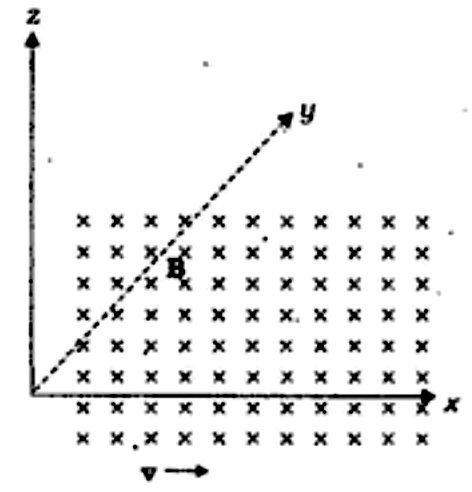Home/Class 12/Physics/

If the magnetic field is parallel to the positive  $$y-$$axis and the charged particle is moving along the positive  $$x-$$axis $$($$Fig.$$).$$ Which way would the Lorentz force be for an electron $$($$negative charge$$).$$Speed
00:00
02:44## QuestionPhysicsClass 12

If the magnetic field is parallel to the positive  $$y-$$axis and the charged particle is moving along the positive  $$x-$$axis $$($$Fig.$$).$$ Which way would the Lorentz force be for an electron $$($$negative charge$$).$$

See analyssi below
4.64.6## SolutionAccording to screw rule or right-hand thumb rule we can say that, the velocity  $$v$$  of particle is along the  $$x-$$ axis. While  $$B$$  the magnetic field is along the  $$y-$$axis. So  $$v \times B$$ is along the  $$z-$$axis. So, for electron it will be along  $$z-$$axis.